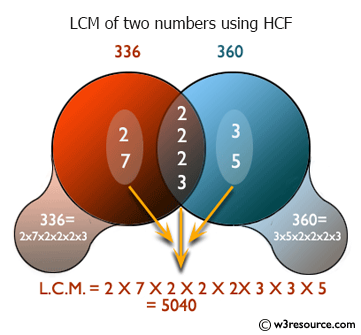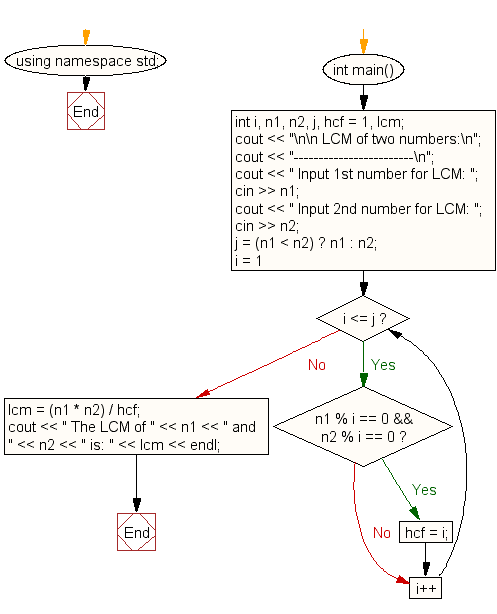﻿ C++ Exercises: Find LCM of any two numbers using HCF - w3resource# C++ Exercises: Find LCM of any two numbers using HCF

## C++ For Loop: Exercise-29 with Solution

Write a program in C++ to find LCM of any two numbers using HCF.

Pictorial Presentation:Sample Solution:-

C++ Code :

``````#include <iostream>
using namespace std;

int main()
{
int i, n1, n2, j, hcf = 1, lcm;
cout << "\n\n LCM of two numbers:\n";
cout << "------------------------\n";
cout << " Input 1st number for LCM: ";
cin >> n1;
cout << " Input 2nd number for LCM: ";
cin >> n2;
j = (n1 < n2) ? n1 : n2;
for (i = 1; i <= j; i++) {

if (n1 % i == 0 && n2 % i == 0) {
hcf = i;
}
}
/* mltiplication of HCF and LCM = the multiplication of these two numbers.*/
lcm = (n1 * n2) / hcf;
cout << " The LCM of " << n1 << " and " << n2 << " is: " << lcm << endl;
}
``````

Sample Output:

``` LCM of two numbers:
------------------------
Input 1st number for LCM: 15
Input 2nd number for LCM: 25
The LCM of 15 and 25 is: 75
```

Flowchart:C++ Code Editor:

Contribute your code and comments through Disqus.

What is the difficulty level of this exercise?

﻿

## C++ Programming: Tips of the Day

What is a smart pointer and when should I use one?

This answer is rather old, and so describes what was 'good' at the time, which was smart pointers provided by the Boost library. Since C++11, the standard library has provided sufficient smart pointers types, and so you should favour the use of std::unique_ptr, std::shared_ptr and std::weak_ptr.

There was also std::auto_ptr. It was very much like a scoped pointer, except that it also had the "special" dangerous ability to be copied - which also unexpectedly transfers ownership.

It was deprecated in C++11 and removed in C++17, so you shouldn't use it.

```std::auto_ptr<MyObject> p1 (new MyObject());
std::auto_ptr<MyObject> p2 = p1; // Copy and transfer ownership.
// p1 gets set to empty!
p2->DoSomething(); // Works.
p1->DoSomething(); // Oh oh. Hopefully raises some NULL pointer exception.
```

Ref : https://bit.ly/3mc9GHE